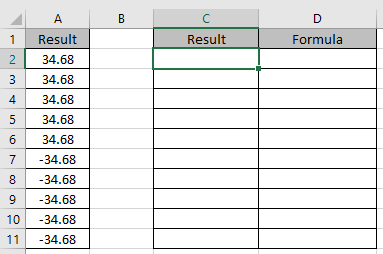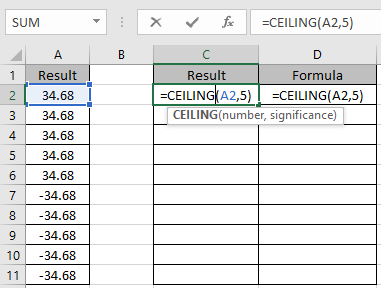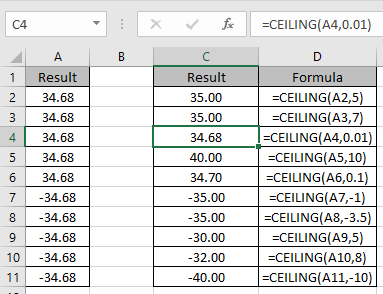# How to use the Excel CEILING function

CEILING function is categories as Math or Trig function in excel returns a number rounded up based on a multiple of significance.

In simple words, Excel CEILING function works just like MROUND function in Excel. This function always rounds up to the significant number
Syntax

=CEILING (number, Significance)

Number: Number to be rounded up.
Significance: Rounded up to a number.

Let’s use this function on the data range shown below.Here We have 5 positive values and 5 negative values. We will be using different significant numbers.
Use the formula:

=CEILING(A2, 5)Copy the formula in rest of cells to get the whole output using Ctrl + DAs you can see we got different numbers as output because the significance number always varied.

Hope you understood how to use the CEILING function to round the number in Excel. Explore more articles on Excel function here. Please feel free to state your query or feedback for the above article.

Related Articles:

How to Use the FLOOR.MATH Function in Excel

Formula Round to nearest 100 and 1000

How to Use Round function in Excel

How to Use RoundUp function in Excel

How to Use Rounddown function in Excel

Rounding Numerical Calculation Results In Microsoft Excel

Popular Articles:

50 Excel Shortcuts to Increase Your Productivity

How to use the VLOOKUP Function in Excel

How to use the COUNTIF function in Excel 2016

How to Use SUMIF Function in Excel

Terms and Conditions of use

The applications/code on this site are distributed as is and without warranties or liability. In no event shall the owner of the copyrights, or the authors of the applications/code be liable for any loss of profit, any problems or any damage resulting from the use or evaluation of the applications/code.